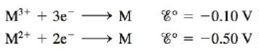Chapter 18, Problem 150CP

Chapter
Section
Textbook Problem

# Given the following two standard reduction potentials,solve for the standard reduction potential of the half-reaction M 3 + +   e −   →   M 2 + (Hint: You must use the extensive property ∆G° to determine the standard reduction potential.)

Interpretation Introduction

Interpretation:

The standard reduction potential of two half-cells is given. The standard reduction potential for the given half-reaction is to be calculated.

Concept introduction:

The standard reduction potential is the potential of the cell at the standard conditions like 1.0M concentration and a pressure of 1atm at the given temperature conditions.

To determine: The standard reduction potential of the given half-cell reaction.

Explanation

The given reactions are as follows,

M3++3eME°=0.10V (1)

M2++2eME°=0.50V (2)

Subtract both the equations to obtain the given reaction as,

M3++eM2+ (3)

The cell potential for the equation (3) is calculated using the relationship between cell potential and change in Gibbs free energy, ΔG°=nFE° .

Where the following terms are defined as follows,

• ΔG° is the change in Gibbs free energy.
• n is the number of electrons involved in the cell reaction.
• F is the Faraday’s constant (96485C/molee) .
• E° is the cell potential.

The change in Gibbs free energy for equation (1) is calculated as follows,

ΔG°1=-nFE°1=-(3)×96485×(-0.10V)=28,945

### Still sussing out bartleby?

Check out a sample textbook solution.

See a sample solution

#### The Solution to Your Study Problems

Bartleby provides explanations to thousands of textbook problems written by our experts, many with advanced degrees!

Get Started